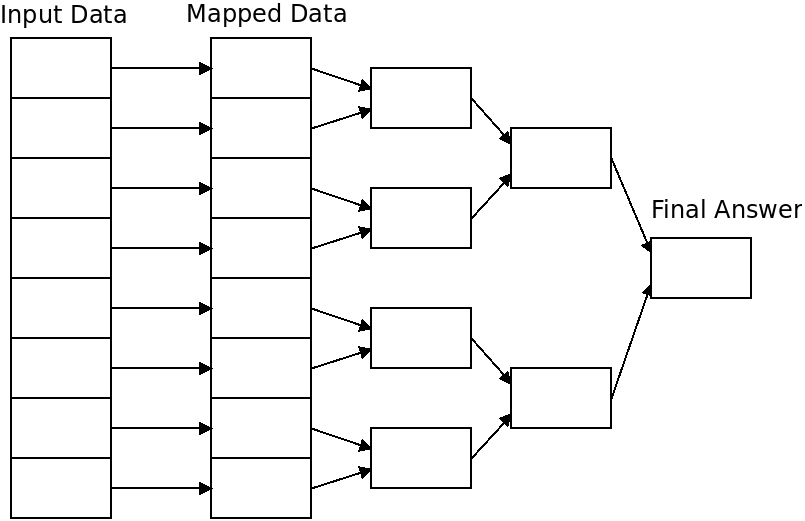# Parallel Algorithms

## Overview

Algorithms vary greatly in how effectively they can be parallelized. Some, such as the knapsack solver, are quite easy to parallelize as they contain little need to communicate between tasks. These are called "embarrassingly parallel" problems. Embarrassingly parallel algorithms typically do not have problems achieving linear speedup.

Other algorithms can be parallelized in part, but require communication which makes them more difficult to implement correctly, and limits the speedup.

Other problems have no known efficient parallel algorithm. These problems include the $P$-complete set of problems, which are the most difficult problems which can be solved in polynomial time.

Today we will talk about a few approaches to writing parallel algorithms.

## Map Reduce

One class of algorithms that can be parallelized effectively are MapReduce algorithms. A MapReduce takes two parts:

• Map

This does a transformation of some kind on a piece of data. Multiple threads or processes can do this transformation on their own data in parallel.

• Reduce

This combines the results of all of the transformed data into one final result. These can be parallelized using the tree exchange approach discussed earlier.

Because the map step can be parallelized linearly, and the reduce step takes $O(log N)$ steps with $N$ nodes, MapReduce is very effective at speeding up sequential tasks.## Map Reduce Example

Many problems can be solved using this technique. For instance, the task of finding the integer with the longest Collatz sequence can be solved with MapReduce.

Here the "map" step is to transform the integer into the number of steps it takes to reach 1.

The "reduce" step is to find the largest value from the previous step.## Map Reduce Applications

Many other problems can be solved with MapReduce algorithms:

• Finding the sum/product/min/max of a set of numbers.
• Counting the number of times an element appears in a set of inputs.
• Searching.

As we have seen, MPI and OpenMP include automatic reduce functionality, and writing MapReduce programs in any parallel system is fairly straightforward.

Systems like Hadoop facilitate writing distributed MapReduce programs, where you essentially only need to write the map function, and specify what reduction to use.

Additionally, some databases provide the ability to run parallel MapReduce queries on the data stored in tables.

## Grid Algorithms

Another widespread approach to parallel computing is to break the input data into a grid wherein each processor or thread owns some portion of the data. This is the approach taken by the image blur and forest fire assignments.

Other uses of this approach include:

• Computational dynamics.
• Simulations.
• Image processing.
• Computer vision.
• Computational geometry.

## Divide and Conquer Algorithms

Divide and conquer algorithms are those which divide a problem into portions, and then solve each portion recursively. An example is the merge sort algorithm, which is given below:

1. If the array has 0 or 1 items, we're done.
2. Mergesort the left half.
3. Mergesort the right half.
4. Merge the two halves together to make a new, sorted, array.

Which portion of this algorithm can be parallelized?

Another parallelizeable divide and conquer algorithm is the Fourier transform which is widely used in digital signal processing.